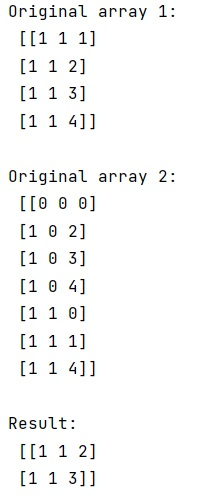# Remove all elements contained in another array

Learn, how can we remove all elements contained in another array in Python? By Pranit Sharma Last updated : March 31, 2023

Suppose that we are given two numpy arrays which contain some common elements and we need to delete those elements from the first array that are present in the first array.

## Removing elements contained in another array

To remove all elements contained in another array, we need to find the row indices of several values that are in another array. We can do this using in1d() and if the arrays are multi-dimensional, we can flatten the arrays using ravel() and then we can remove the matching elements from array 1.

Let us understand with the help of an example,

## Python program to remove all elements contained in another array

```# Import numpy
import numpy as np

# Creating numpy arrays
arr1 = np.array([[1, 1, 1],
[1, 1, 2],
[1, 1, 3],
[1, 1, 4]])

arr2 = np.array([[0, 0, 0],
[1, 0, 2],
[1, 0, 3],
[1, 0, 4],
[1, 1, 0],
[1, 1, 1],
[1, 1, 4]])

# Display Original arrays
print("Original array 1:\n",arr1,"\n")
print("Original array 2:\n",arr2,"\n")

# Getting row indices of common elements and removing them
size = np.maximum(arr2.max(0),arr1.max(0))+1
res = arr1[~np.in1d(np.ravel_multi_index(arr1.T,size),np.ravel_multi_index(arr2.T,size))]

# Display result
print("Result:\n",res,"\n")
```

### Output